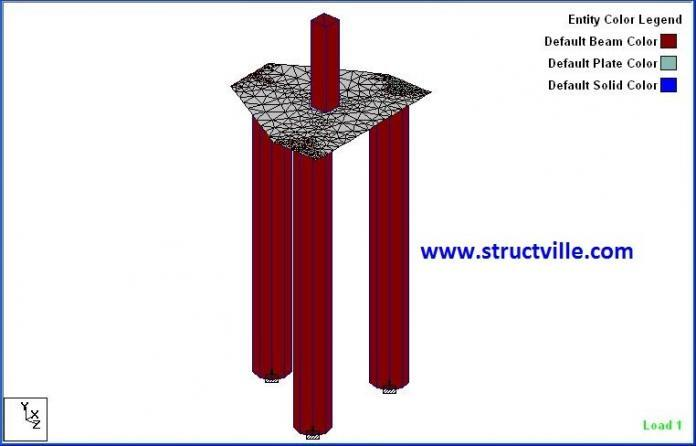In our post on Structural Aspects of Pile Foundation Design, we showed how we can determine the number piles required to support a column load, in which we went ahead to design a triangular pile cap based on strut and tie method (truss analogy). In this post, we are going to design the same triangular pile cap using bending theory and finite element analysis from Staad Pro.

The layout of the pile cap is shown Figure 1 and we are going to assume that it is subjected to an axial load of 2500 kN from a 300mm x 300mm column (neglect the orientation of the column in Figure 1).

(a) Analysis Using Bending Theory
The load on each pile is computed based on its distance from the centroid of the column

N1 = N(Ay – Ay‘)/Ay
Where:
N = Axial load from column = 2500 kN
Ay = Vertical distance between the centriod of piles in the y-direction = 515 + 1045 = 1560 mm
Ay‘ = Vertical distance between the centriod of column to centroid of single pile in the y-direction = 1045 mm

Hence N1 = 2500 x (1560 – 1045)/1560 = 825. 321 kN

N2 = (N – N1 ) / 2
N2 = (2500 – 825.321)/2 = 837.3395 kN

Bending moment in the x-direction
Mx = N2 x (Ax – Cx)/2
Ax = Spacing of the twin piles = 1800 mm
Cx = Width of the column parallel to x-direction = 300 mm

Mx = 837.3395 x (1.8 – 0.3)/2 = 628 kNm

Bending moment in the y-direction
My = N1 x (Ay‘ – Cy/2)
Ay‘ = Spacing of the twin piles = 1045 mm
Cy = Width of the column parallel to y-direction = 300 mm
My = 825. 321 x (1.045 – 0.3/2) = 738.662 kNm

(b) Analysis using Finite Element Analysis
The pile cap was modelled as a 1300 mm thick concrete plate element on Staad Pro, while the piles were modelled as 600 mm diameter columns with a length of 2m supported on a fixed base. The short column was adopted to avoid wide variation of result due to second order effects and to avoid large displacement which is not practicable and also not considered in manual analysis.

The results obtained from Staad Pro are given in Figures 3 – 6 below;

The summary of the results between manual analysis (bending theory) and Staad Pro (finite element analysis) is shown in Table 1.

### Comparison Table

Table 1: Comparison of results between manual bending theory and finite element analysis

From Table 1, it could be seen that the distribution of load to the piles was found to be approximately the same for both approaches, but a wide variation was observed for Mx bending moment between the two methods. The My bending moment was found to be close with a percentage difference of about 3.229%. Therefore, Staad Pro can be used to model triangular pile caps and the result can be used for design purposes. The result obtained from this study is open to discussion, especially based on the difference obtained in bending moment values. We will be glad to hear from you!

1.GOWTHAMI V V